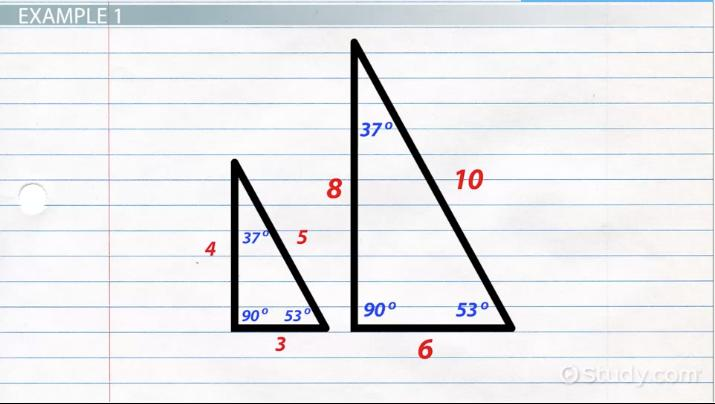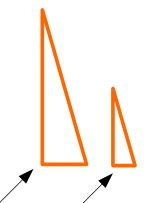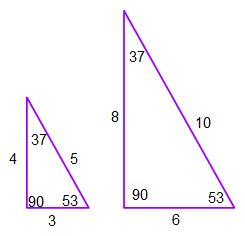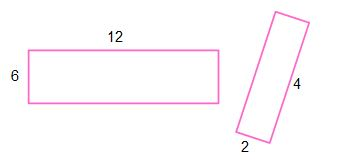# Similarity Transformations in Corresponding Figures

Lesson Transcript
Instructor: Yuanxin (Amy) Yang Alcocer

Amy has a master's degree in secondary education and has been teaching math for over 9 years. Amy has worked with students at all levels from those with special needs to those that are gifted.

Similarity transformations in corresponding figures can be used to compare similar shapes of different sizes. Look at two corresponding figures and discover how to determine if they are similar before analyzing two examples. Updated: 11/11/2021

## Two Figures

Imagine you're a famous artist. You've been contracted by your city to build two sets of similar figures in front of the city hall for a modern art installation. The city wants to have a pair of similar triangles and a pair of similar rectangles. In math, we say that two figures are similar when the shapes are the same with the only difference being the size.

For example, a model car is similar to the real life car that it models. A model house is similar to the real life house that it models. If the model is built to scale, it has the same proportions as the real life object; the only way you can tell them apart is that they are different sizes. Even if one object was rotated a different way, you could still see that they look the same except for the size.An error occurred trying to load this video.

Try refreshing the page, or contact customer support.

Coming up next: How to Prove Relationships in Figures using Congruence & Similarity

### You're on a roll. Keep up the good work!

Replay
Your next lesson will play in 10 seconds
• 0:00 Two Figures
• 0:48 Are They Similar?
• 2:50 Example 1
• 4:21 Example 2
• 6:30 Lesson Summary
Save Save

Want to watch this again later?

Timeline
Autoplay
Autoplay
Speed Speed

## Are They Similar?

As the artist for the city now, you need to recognize when two figures are similar. You're looking at a pair of triangles and a pair of rectangles that you've made. What are the criteria for checking to see if two figures are similar? There are two.

The first is that the corresponding angles are all congruent to each other. Corresponding means they are located at the same location if the two figures are placed in the same way. For example, the right angles of two right triangles are corresponding angles. Even if you rotated one triangle, that right angle is still the corresponding angle to the other right angle.The second criterion is that all the corresponding sides are proportional to each other. This means that if one pair of corresponding sides has a ratio of 2:1, meaning that one figure is twice as large as the other, then all the other corresponding sides will have the same ratio. Always remember to simplify these ratios as you would fractions.

You can see that it's not hard to spot corresponding angles and corresponding sides when the figures are placed in the same way, but what if the figures are arranged differently? For example, it may be a little more difficult to identify corresponding parts between two triangles when one triangle is rotated differently, flipped upside down, or even just far away from the other triangle.

Luckily, we have transformations, or ways to rearrange a figure. There are several transformations to help us place our figures in the same way. You can use rotation to turn one figure until it's placed in the same way as the other figure. If one of your figures were upside down, then you can use a reflection, or flip it across a line, to get it right side up. If one figure is far away from the other, perform a translation, or slide the figure until it's closer. All three of these transformations won't change the size or shape of the figure, so you'll still have all of your corresponding angles and corresponding sides if the two figures are similar.

Now that you know how to use transformations to place your figures in the same way, let's see how you can figure out if your two triangles and two rectangles are similar.

## Example 1

The pair of triangles that you are looking at are these two.No transformations (no rotations, no reflections, and no translations) are needed here, as both triangles are facing the same way. You can easily see the corresponding angles and sides. The top angles are corresponding angles. The bottom left angles are corresponding and so are the bottom right angles of each triangle. The bottom sides of each triangle are corresponding. The left straight edge sides are corresponding. And, the slanted edges are corresponding.

In order for these two triangles to be similar, all the corresponding angles have to be congruent. They have to be the same. All the corresponding sides must be proportional. You look at the corresponding angles of your two triangles first. Are they are all the same? Yes, they are. You have 90 and 90, 37 and 37, and 53 and 53. This part checks off.

Next, you look at your corresponding sides. Are they are all proportional by the same amount? Let's see. Starting with the bottom side and working your way clockwise, you get 6:3, which simplifies to 2:1. The next side, the straight side on the left, gives you a ratio of 8:4, which simplifies to 2:1. Last, the slanted edge gives you a ratio of 10:5, which also simplifies to 2:1. Keeping your pattern of larger triangle to smaller triangle, you see that the ratios for each set of corresponding sides are all the same. This means that your two triangles are similar because they have congruent corresponding angles and proportional corresponding sides.

## EXAMPLE 2

Now, let's check the two rectangles. Are these similar?To unlock this lesson you must be a Study.com Member.

### Register to view this lesson

Are you a student or a teacher?